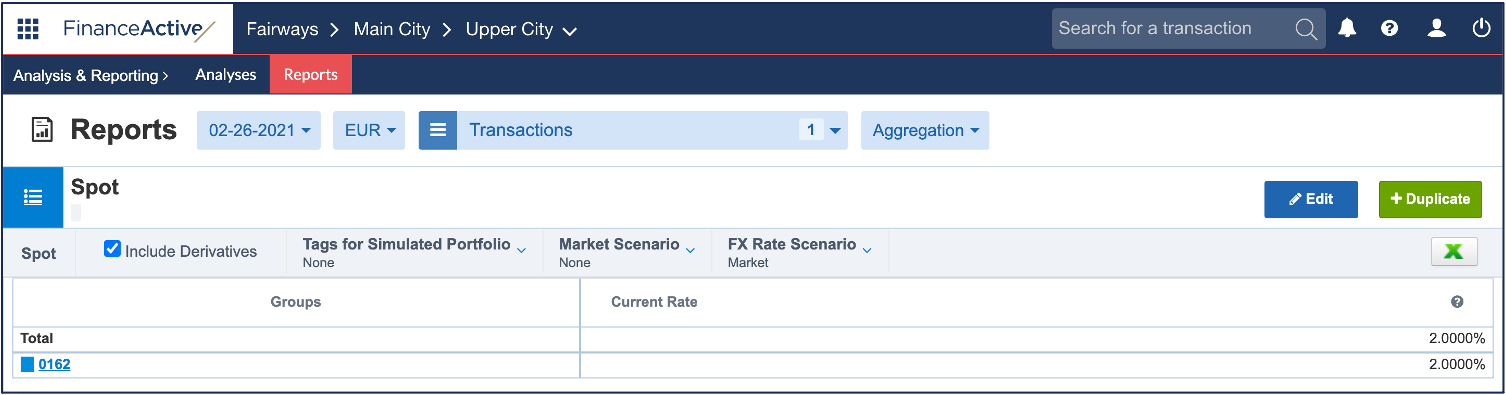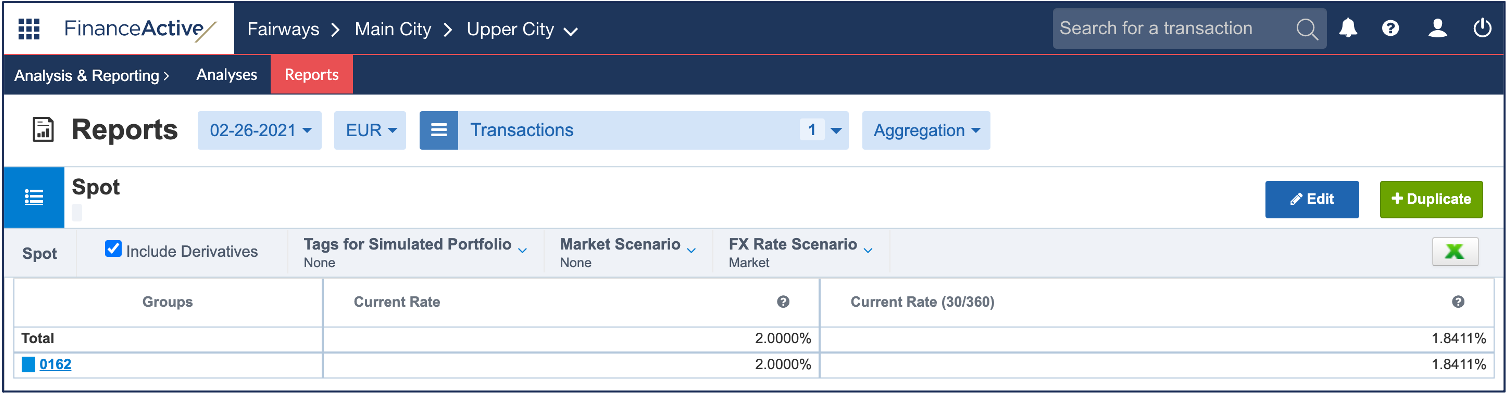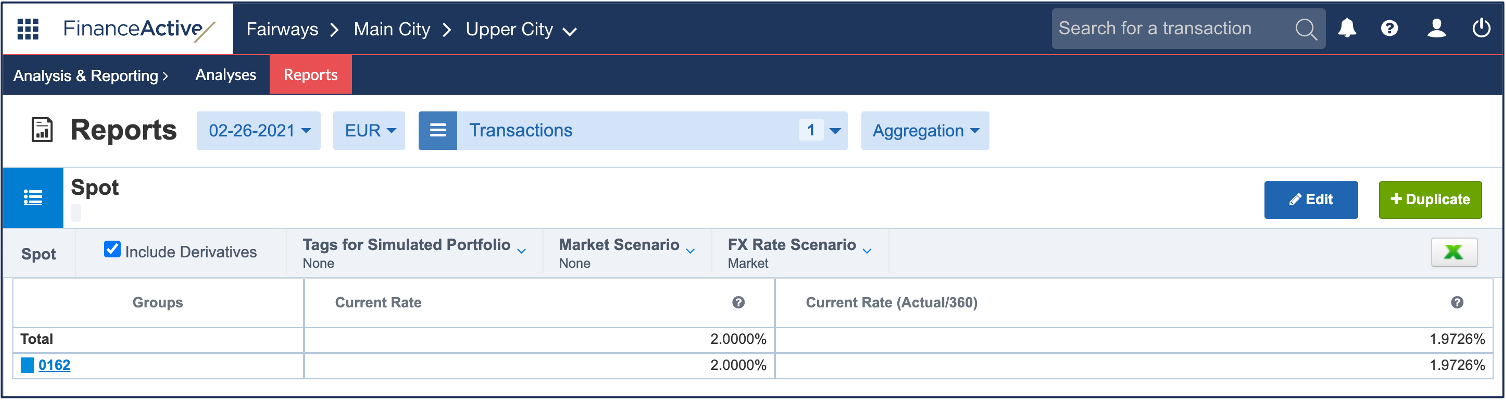# Rate (current)

The current rate in Fairways Debt is the interest rate of the current period of the transaction. This rate can be converted into a uniform interest rate method (30/360, Actual 30/360, etc.).

## Spot Reports

Rate (current)

Interest rate of the current period of the transaction.

In this example, the current rate of 0162 on 26 February 2021 is 2%.• 26 February 2021 is included in the 1 February 2021 - 1 March 2021 period.
• The 1 February 2021 - 1 March 2021 period is associated with an interest rate of 2%.Rate (current, 30/360)

Interest rate of the current period of the transaction, converted into 30/360 US, using an average of each equivalent current rate, weighted by the outstanding balance, and taking into account derivatives.Rate (current, actual/360)

Interest rate of the current period of the transaction, converted into Actual/360, using an average of each equivalent current rate, weighted by the outstanding balance, and taking into account derivatives.